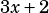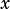Hi! On q8 page 149 (polynomials), what is happening in D? Why is it true?

This question (image below) asks which statement is FALSE. Generally, the best way to approach a question like this is to evaluate the simplest answer choices first and see if you can find one that’s false. If you do that, you probably never have to try D since B is much simpler to test and (spoiler alert) turns out to be false. But since you’re asking what’s going on in D, see below.

Choice D defines a new function,. Let’s figure out what that looks like.Now, is that divisible by? Well, if it were, then it should have a zero when, right? Which means it’d have a zero at. Does it?

As it turns out, yes! You can see this quickly by graphing, or by pluggingin forthroughout the equation: